# Parallel Programming in the Cloud with Python Dask

I am always looking for better ways to write parallel programs.  In chapter 7 of our book “Cloud Computing for Science and Engineering” we looked at various scalable parallel programming models that are used in the cloud.   We broke these down into five models: (1) HPC-style “Single Program Multiple Data” (SPMD) in which a single program communicates data with copies of itself running in parallel across a cluster of machines, (2) many task parallelism that uses many nearly identical workers processing independent data sets, (3) map-reduce and bulk synchronous parallelism in which computation is applied in parallel to parts of a data set and intermediate results of a final solution are shared at well defined, synchronization points,  (4) graph dataflow transforms a task workflow graph into sets of parallel operators communicating according to the workflow data dependencies and (5) agents and microservices  in which a set of small stateless services process incoming data messages and generate messages for other microservices to consume.  While some applications that run in the cloud are very similar to the batch style of HPC workloads, parallel computing in the cloud is often driven by different classes application requirements.  More specifically, many cloud applications require massive parallelism to respond external events in real time.  This includes thousands of users that are using apps that are back-ended by cloud compute and data.   It also includes applications that are analyzing streams of data from remote sensors and other instruments.   Rather than running in batch-mode with a start and end, these applications tend to run continuously.

A second class of workload is interactive data analysis.   In these cases, a user is exploring a large collection of cloud resident data.   The parallelism is required because the size of the data: it is too big to download and if you could the analysis would be too slow for interactive use.

We have powerful programming tools that can be used for each of the parallel computing models described above but we don’t have a single programming tool that support them all.   In our book we have used Python to illustrate many of the features and services available in the commercial clouds.  We have taken this approach because Python and Jupyter are so widely used in the science and data analytics community.  In 2014 the folks at Continuum (now just called Anaconda, Inc) and a several others released a Python tool called Dask which supports a form of parallelism similar to at least three of the five models described above.  The design objective for Dask is really to support parallel data analytics and exploration on data that was too big to keep in memory.   Dask was not on our radar when we wrote the drafts for our book,  but it certainly worth discussing now.

This is not intended as a full Dask tutorial.   The best tutorial material is the on-line YouTube videos of talks by Mathew Rocklin from Anaconda.   The official  tutorials from Anaconda are also available.  In the examples we will discuss here we used three different Dask deployments.  The most trivial (and the most reliable) deployment was a laptop installation.  This worked on a Windows 10 PC and a Mac without problem.  As Dask is installed with the most recent release of Anaconda, simply update your Anaconda deployment and bring up a Jupyter notebook and “import dask”.    We also used the same deployment on a massive Ubuntu linux VM on a 48 core server on AWS.  Finally, we deployed Dask on Kubernetes clusters on Azure and AWS.

Our goal here is to illustrate how we can use Dask to illustrate several of the cloud programming models described above.    We begin with many task parallelism, then explore bulk synchronous and a version of graph parallelism and finally computing on streams.  We say a few words about SPMD computing at the end, but the role Dask plays there is very limited.

## Many Task Parallelism and Distributed Parallel Data Structures

Data parallel computing is an old important concept in parallel computing.  It describes a programming style where a single operation is applied to collections of data as a single parallel step. A number of important computer architectures supported data parallelism by providing machine instructions that can be applied to entire vectors or arrays of data in parallel.  Called Single instruction, multiple data (SIMD) computers, these machines were the first supercomputers and included the Illiac IV and the early Cray vector machines.  And the idea lives on as the core functionality of modern GPUs.   In the case of clusters computers without a single instruction stream we traditionally get data parallelism by distributed data structures over the memories of each node in the cluster and then coordinating the application of the operation in a thread on each node in parallel.   This is an old idea and it is central to Hadoop, Spark and many other parallel data analysis tools.   Python already has a good numerical array library called numpy, but it only supports sequential operations for array in the memory of a single node.

Dask computations are carried out in two phases.   In the first phase the computation is rendered into a graph where the nodes are actual computations and the arcs represent data movements.   In the second phase the graph is scheduled to run on a set of resources.  This is illustrated below.  We will return to the details in this picture later.Figure 1.  Basic Dask operations: compile graph and then schedule on cluster

There are three different sets of “resources” that can be used.   One is a set of threads on the host machine.   Another is a set of process and the third is a cluster of machines.   In the case of threads and local processes the scheduling is done by the “Single machine scheduler”.   In the case of a cluster it called the distributed cluster.  Each scheduler consumes a task graph and executes it on the corresponding host or cluster.   In our experiments we used a 48 core VM on AWS for the single machine scheduler. In the cluster case the preferred host is a set of containers managed by Kubernetes.   We deployed two Kubernetes clusters:  a three node cluster on Azure and a 6 node cluster on AWS.

Python programmers are used to numpy arrays, so Dask takes the approach to distributing arrays by maintaining as much of the semantics of numpy as possible.  To illustrate this idea consider the following numpy computation that creates a random 4 by 4 array, then zeros out all elements lest than 0.5 and computes the sum of the array with it’s transpose.

```x = np.random.random((4,4))
x[x<0.5] = 0
y = x+x.T
```

We can use Dask to make a distributed version of the same matrix and perform the same computations in parallel.

```Import dask.array as da
x = da.random.random(size = (4,4), chunks =(4,1))
x[x<0.5] = 0
y = x+x.T
```

The important new detail here is that we give explicit instructions on how we want the array to be distributed by specifying the shape of the chunks on each node.   In this case we have said we want each “chunk” to be a 4×1 slice of the 4×4 array.   We could have partitioned it into square blocks of size 2×2.   Dask takes care of managing each chunk and the needed communication between the processes that handle each chunk.   The individual chunks are managed on each thread/process/worker as numpy arrays.

As stated above, there are two parts to a dask computation.   The first phase is the construction of a graph representing the computation involving each chunk. We can actually take a look at the graph.   For example, in the computation above we can use the “visualize()” method as follows.

```y = x+x.T
y.visualize()
```Figure 2.   Sample Dask Graph for x+x.T

The nodes represent data or operations and the lines are data movements from one node to another.  As can be seen this is a rather communication intensive graph.   This is becase the transpose operation requires element on the rows (which are distributed) must be moved to columns on the appropriate node to do the addition.  The way we chunck the array can have a huge impact on the complexity of the distributed computation.  For example, 2×2 chuncking makes this one very easy.   There are 4 chunks and doing the transpose involves only a simple swap of the “off diagonal” chunks.   In this case the graph is much simpler (and easier to read!)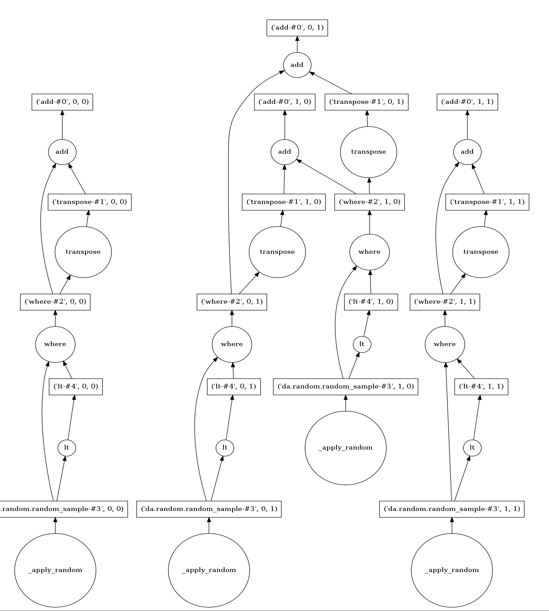Figure 3.  Task graph for x+x.T with 2×2 chunking of data

The second step for Dask is to send the graph to the scheduler to schedule the subtasks and execute them on the available resources. That step is accomplished with a call to the compute method.

```y.compute()
```

Dask arrays support almost all the standard numpy array operations except those that involve complex communications such as sorting.

In addition to numpy-style arrays, Dask also has a feature called Dask dataframes that are distributed versions of Pandas dataframes.   In this case each Dask dataframe is partitioned by blocks of rows where each block is an actual Pandas dataframe.  In other words, Dask dataframes operators are wrappers around the corresponding Pandas wrappers in the same way that Dask array operators are wrappers around the corresponding numpy array operators.    The parallel work is done primarily by the local Pandas and Numpy operators working simultaneously on the local blocks and this is followed by the necessary data movement and computation required to knit the partial results together.  For example, suppose we have a dataframe, df, where each row is a record consisting of a name and a value and we would like to compute the sum of the values associated with each name.   We assume that names are repeated so we need to group all records with the same name and then apply a sum operator.  We set this up on a system with three workers.  To see this computational graph we write the following.

```df.groupby(['names']).sum().visualize()
```Figure 4.  Dataframe groupby reductionFigure 5.  Processing Yellow Cab data for New York City

The persist method moves the dataframe into memory as a persistent object that can be reused without being recomputed.  (Note:  the read_cvs method did not work on our kubernetes clusters because of a missing module s3fs in the dask container, but it did work on our massive shared memory VM which has 200 GB of memory.)

Having loaded the data we can now follow the dask demo example and compute the best hour to be a taxi driver based on the fraction of tip received for the ride.Figure 6.  New York City cab data analysis.
As you can see, it is best to be a taxi driver about 4 in the morning.

A more general distributed data structure is the Dask Bag that can hold items of less structured type than array and dataframes.   A nice example http://dask.pydata.org/en/latest/examples/bag-word-count-hdfs.html illustrates using Dask bags to explore the Enron public email archive.

One of the more interesting Dask operators is one that implements a version of the old programming language concept of a future   A related concept is that of lazy evaluation and this is implemented with the dask.delayed function.   If you invoke a function with the delayed operator it simply builds the graph but does not execute it.  Futures are different.    A future is a promise to deliver the result of a computation later.  The future computation begins executing but the calling thread is handed a future object which can be passed around as a proxy for the result before the computation is finished.

The following example is a slightly modified version of one of the demo programs.   Suppose you have four functions

```def foo(x):
return result
def bar(x):
return result
def linear(x, y):
return result
def three(x, y, z):
return result
```

We will use the distributed scheduler to illustrate this example. We first must create a client for the scheduler. Running this on our Azure Kubernetes cluster we get the following.

```
c = Client()
c
```To illustrate the delayed interface, let us build a graph that composes our example functions

```from dask import visualize, delayed
i = 3
x = delayed(foo)( I )
y = delayed(bar)( x )
z = delayed(linear)(x, y)
q = delayed(three)( x, y, z)
q.visualize(rankdir='LR')
```

In this example q is now a placeholder for the graph of a delated computation.   As with the dask array examples, we can visualize the graph (plotting it from Left to Right).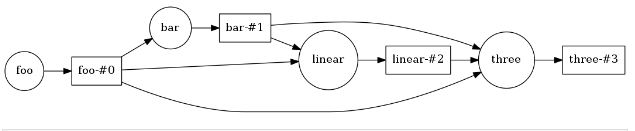Figure 7.  Graph of a delayed computation.

A call to compute will evaluate our graph.   Note that we have implemented the  four functions each with about 1 second of useless computational math (computing the sum of a geometric series) so that we can measure some execution times.   Invoking compute on our delayed computation gives us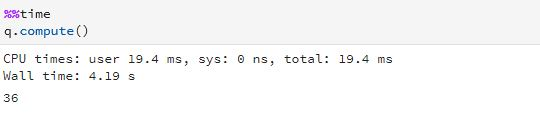which shows us that there is no parallelism exploited here because the graph has serial dependences.

To create a future, we “submit” the function and its argument to the scheduler client.  This immediately returns a reference to future value and starts the computation.  When you need the result of the computation the future has a method “result()” that can be invoked and cause the calling thread to wait until the computation is done.

Now let us consider the case where the we need to evaluate this graph on 200 different values and then sum the results.   We can use futures to kick off a computation for each instance and wait for them to finish and sum the results.   Again, following the example in the Dask demos, we ran the following on our Azure Kubernetes cluster: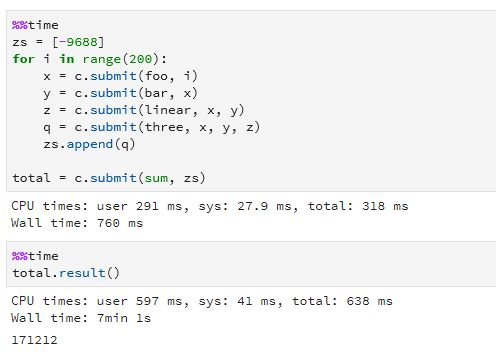Ignore the result of the computation (it is correct). The important result is the time. Calculating the time to run this sequentially (200*4.19 = 838 seconds) and dividing by the parallel execution time we get a parallel speed-up of about 2, which is not very impressive. Running the same computation on the AWS Kubernetes cluster we get a speed-up of 4. The Azure cluster has 6 cores and the AWS cluster has 12, so it is not surprising that it is twice as fast. The disappointment is that the speed-ups are not closer to 6 and 12 respectively.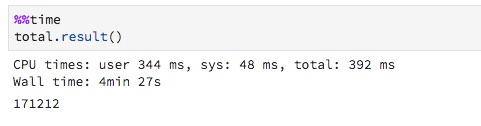Results with AWS Kubernetes Cluster

However, the results are much more impressive on our 48 core AWS virtual machine.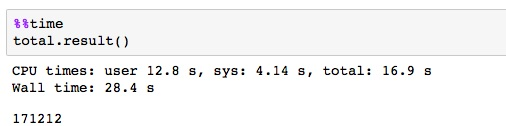Results with AWS 48-core VM

In this case we see a speed-up of 24.   The difference is the fact that the scheduling is using shared memory and threads.

Dask futures are a very powerful tool when used correctly.   In the example above, we spawned off 200 computations in less than a second.   If the work in the individual tasks is large, that execution time can mask much of the overhead of scheduler communication and the speed-ups can be much greater.

Dask has a module called streamz that implements a basic streaming interface that allows you to compose graphs for stream processing.   We will just give the basic concepts here.   For a full tour look at https://streamz.readthedocs.io.   Streamz graphs have sources,  operators and sinks.   We can start by defining some simple functions as we did for the futures case:

```def inc(x):
return x+13
def double(x):
return 2*x
def fxy(x): #expects a tuple
return x+ x
return x+y
from streamz import Stream
source = Stream()
```

The next step will be to create a stream object and compose our graph.   We will describe the input to the stream later.   We use four special stream operators here.    Map is how we can attach a function to the stream.   We can also merge two streams with a zip operator.   Zip waits until there is an available object on each stream and then creates a tuple that combines both into one object.   Our function fxy(x) above takes a tuple and adds them.   We can direct the output of a stream to a file, database, console output or another stream with the sink operator.  Shown below our graph has two sink operators.Figure 8.  Streamz stream processing pipeline.

Visualizing the graph makes this clear.   Notice there is also an accumulate operator.   This allows state flowing through the stream to be captured and retained.   In this case we use it to create a running total.  To push  something into the stream we can use the emit() operator as shown below.The emit() operator is not the only way to send data into a stream. You can create the stream so that it takes events from kafka, or reads lines from a file or it can monitor a file system directory looking for new items. To illustrate that we created another stream to look at the home director of our kubernetes cluster on Azure. Then we started this file monitor. The names of the that are there are printed. Next, we added another file “xx” and it picked it up. Next, we invoked the stream from above and then added another file “xxx”.# Handling Streams of Big Tasks

Of the five types of parallel programming Dask covers 2 and a half:  many task parallelism, map-reduce and bulk synchronous parallelism and part of graph dataflow.   Persistent microservices  are not part of the picture.   However, Dask and Streamz can be used together to handle one of the use cases for microservices.  For example, suppose you have a stream of tasks and you need to do some processing on each task but the arrival rate of tasks exceed the rate at which you can process them.   We treated this case with Microservices while processing image recognition with MxNet and the resnet-152 deep learning model (see this article.)  One can  use the Streams sink operation to invoke a future to spawn the task on the Kubernetes  cluster.   As the tasks finish the results can be pushed to other processes for further work or to a table or other storage as illustrated below.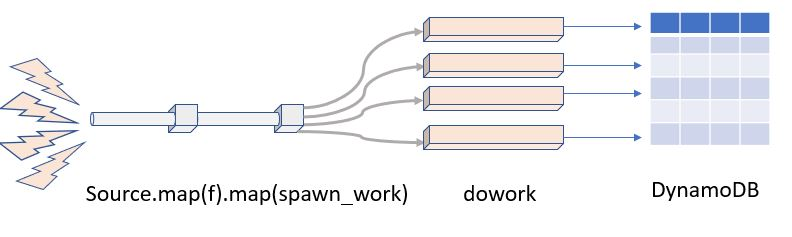Figure 9 Extracting parallelism from a stream.

In the picture we have a stream called Source which gathers the events from external sources.  We then map it to a function f() for initial processing. The result of that step is sent to a function called spawn_work which creates a future around a function that does some deep processing and sends a final result to an AWS DynamoDB table.   (The function putintable(n) below shows an example.  It works by invoking a slow computation then create the appropriate DynamoDB metadata and put the item in the table “dasktale”.)

```def putintable(n):
import boto3
e = doexp(n*1000000)
dyndb = boto3.resource('dynamodb', … , region_name='us-west-2' )
table.put_item(Item= item )
return e

def spawn_work(n):
x = cl.submit(putintable, n)
```

This example worked very well. Using futures allowed the input stream to work at full speed by exploiting the parallelism. (The only problem is that boto3 needs to be installed on all the kubernetes cluster processes. Using the 48 core shared memory machine worked perfectly.)
Dask also has a queue mechanism so that results from futures can be pushed to a queue and another thread can pull these results out. We tried as well, but the results were somewhat unreliable.

# Conclusion

There are many more stream, futures, dataframe and bag operators that are described in the documents.   While it is not clear if this stream processing tool will be robust enough to replace any of the other systems current available, it is certainly a great, easy-to-use teaching tool.   In fact, this statement can be made about the entire collection of Dask related tools.   I would not hesitate to use it in an undergraduate course on parallel programming.   And I believe that Dask Dataframes technology is very well suited to the challenge of big data analytics as is Spark.

The example above that uses futures to extract parallelism from a stream challenge is interesting because it is completely adaptive. However, it is essential to be able to launch arbitrary application containers from futures to make the system more widely applicable.   Some interesting initial work has been done on this at the San Diego Supercomputer center using singularity to launch jobs on their resources using Dask.   In addition the UK Met Office is doing interesting things with autoscaling dask clusters.   Dask and StreamZ are still young.   I expect them to continue to evolve and mature in the year ahead.